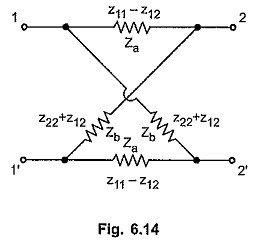## Lattice Network:

The lattice network is one of the common four terminal networks. These networks are generally used in filter sections and also used as symmetrical attenuators.

Consider the lattice network shown in the Fig. 6.12 (a). The arms with impedances Za and Zd are called series arms while the arms with impedances Zb and Zc are called diagonal arms. The lattice network can be redrawn in the form of the bridge network as shown in the Fig. 6.12 (b).

The lattice network is said to be symmetrical if Za = Zd and Zb = Zc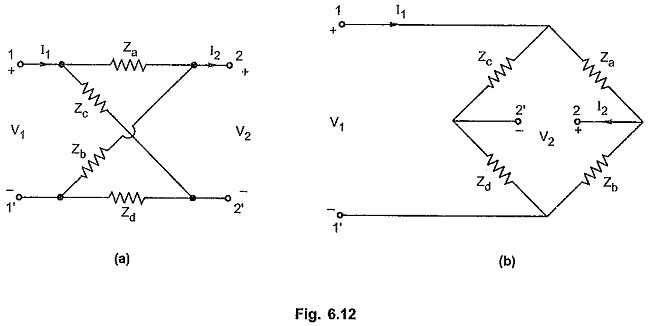Consider I2 = 0 i.e. port 2 open circuited. The network can be redrawn as shown in the Fig. 6.13 (a).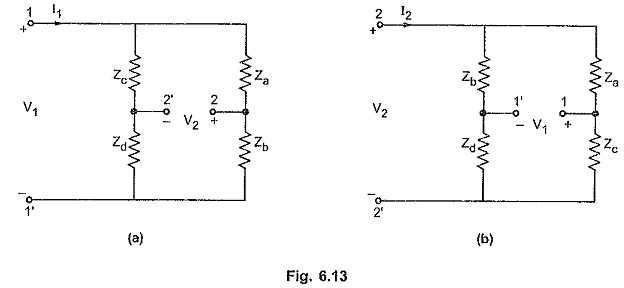Voltage V1 can be expressed interms of current I1 as follows,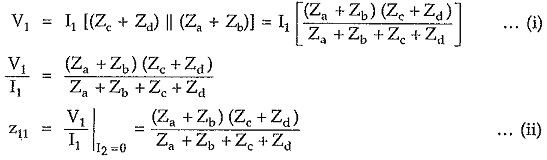The voltage at port 2, V2, can be expressed in terms of current I2 as follows.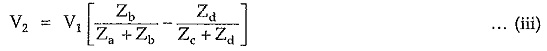Putting value of V1 from equation (i), we get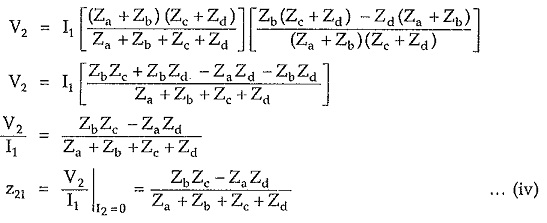Now consider I1 = 0 i.e. port 1 open circuited as shown in the Fig. 6.13 (b).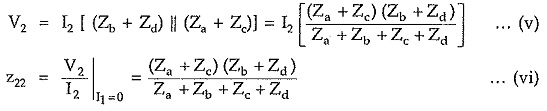Similarly we can express voltage V1 in terms of voltage V2 asPutting value of V2 from equation (v) in above equation, we get,But for symmetrical networks,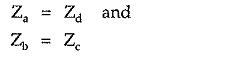Thus equations (ii), (iv), (vi) and (viii) can be written as follows.Hence we can express series arm and diagonal arm impedances in terms of z-parameters of the network as follows,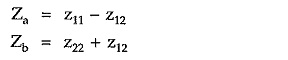Thus, we can express any symmetrical network interms of its lattice equivalent network is the z-parameters of the symmetrical network are known. The lattice network in terms of z-parameters is as shown in the Fig. 6.14.